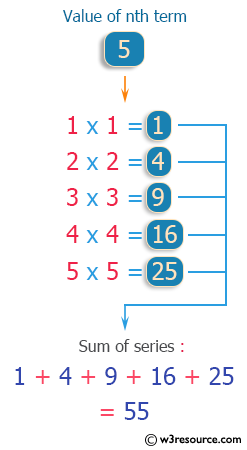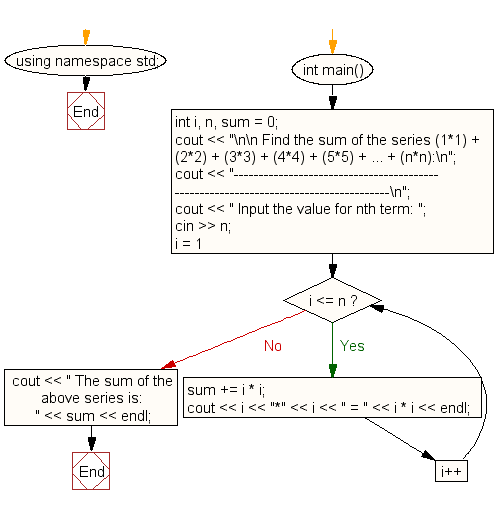﻿ C++ : Calculate the sum of the series (1*1)+(2*2)+.. +(n*n)# C++ Exercises: Calculate the sum of the series (1*1) + (2*2) + (3*3) + (4*4) + (5*5) + ... + (n*n)

## C++ For Loop: Exercise-12 with Solution

Write a program in C++ to calculate the sum of the series (1*1) + (2*2) + (3*3) + (4*4) + (5*5) + ... + (n*n).

Pictorial Presentation:Sample Solution :-

C++ Code :

``````#include <iostream>
using namespace std;

int main()
{
int i, n, sum = 0;
cout << "\n\n Find the sum of the series (1*1) + (2*2) + (3*3) + (4*4) + (5*5) + ... + (n*n):\n";
cout << "------------------------------------------------------------------------------------\n";
cout << " Input the value for nth term: ";
cin >> n;

for (i = 1; i <= n; i++)
{
sum += i * i;
cout << i << "*" << i << " = " << i * i << endl;
}
cout << " The sum of the above series is: " << sum << endl;
}
``````

Sample Output:

``` Find the sum of the series (1*1) + (2*2) + (3*3) + (4*4) + (5*5) + ...
+ (n*n):
-----------------------------------------------------------------------
-------------
Input the value for nth term: 5
1*1 = 1
2*2 = 4
3*3 = 9
4*4 = 16
5*5 = 25
The sum of the above series is: 55
```

Flowchart:C++ Code Editor:

Contribute your code and comments through Disqus.

What is the difficulty level of this exercise?

﻿

## C++ Programming: Tips of the Day

What is a smart pointer and when should I use one?

This answer is rather old, and so describes what was 'good' at the time, which was smart pointers provided by the Boost library. Since C++11, the standard library has provided sufficient smart pointers types, and so you should favour the use of std::unique_ptr, std::shared_ptr and std::weak_ptr.

There was also std::auto_ptr. It was very much like a scoped pointer, except that it also had the "special" dangerous ability to be copied - which also unexpectedly transfers ownership.

It was deprecated in C++11 and removed in C++17, so you shouldn't use it.

```std::auto_ptr<MyObject> p1 (new MyObject());
std::auto_ptr<MyObject> p2 = p1; // Copy and transfer ownership.
// p1 gets set to empty!
p2->DoSomething(); // Works.
p1->DoSomething(); // Oh oh. Hopefully raises some NULL pointer exception.
```

Ref : https://bit.ly/3mc9GHE